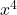Question

Factor.
x^4-9

(x^2-3i) (x^2 + 3i)
(x-3) (x+3)
(x^2 -3) (x^2 + 3)
(x^2 – 3) (x^2 -3)

1.linhdan

(x² – 3)(x² + 3)

Step-by-step explanation:– 9 ← is a difference of squares and factors in general as

a² – b² = (a – b)(a + b) , thus– 9

= (x² )² – 3²

= (x² – 3)(x² + 3)